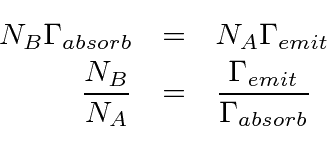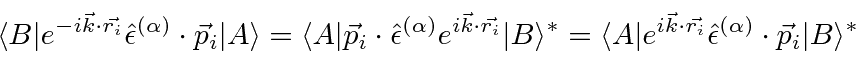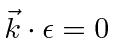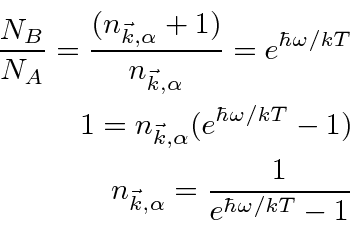## Black Body Radiation Spectrum

We are in a position to fairly easily calculate the spectrum of Black Body radiation. Assume there is a cavity with a radiation field on the inside and that the field interacts with the atoms of the cavity. Assume thermal equilibrium is reached.

Let's take two atomic states that can make transitions to each other:and. From statistical mechanics, we haveand for equilibrium we must haveWe have previously calculated the emission and absorption rates. We can calculate the ratio between the emission and absorption rates per atom:where the sum is over atomic electrons. The matrix elements are closely related.We have used the fact that. The two matrix elements are simple complex conjugates of each other so that when we take the absolute square, they are the same. Therefore, we may cancel them.Now suppose the walls of the cavity are black so that they emit and absorb photons at any energy. Then the result for the number of photons above is true for all the radiation modes of the cavity. The energy in the frequency intervalper unit volume can be calculated by multiplying the number of photons by the energy per photon times the number of modes in that frequency interval and dividing by the volume of the cavity.This was the formula Plank used to start the revolution.

Jim Branson 2013-04-22